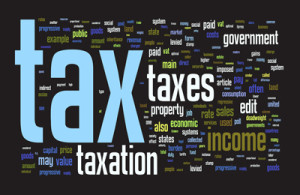### Salary Income Tax Calculator Pakistan 2019-2020How to calculate income tax on salary?
As per the Finance Act 2013 approved by Government of Pakistan, this web based tax calculator applies income tax rates in Pakistan on taxable income of salaried persons and salaried class. A salaried person class slabs are applicable where salary income exceeds more than 50% of the total taxable income. Please share with us your comments and valuable feedback.

Enter Your Monthly Salary Here:

#### Tax Slabs for Salaried Income

Where the salary income individual exceeds seventy-five percent of his taxable income, the rates of tax applied will be as follows:

 S. No Taxable Income Rate of Tax (1) (2) (3) 1. Where the taxable income does not exceed Rs. 600,000 0% 2. Where the taxable income exceeds Rs. 600,000 but does not exceed Rs. 1,200,000 5% of the amount exceeding Rs. 600,000 3. Where taxable income exceeds Rs. 1,200,000 but does not exceed Rs. 1,800,000 Rs. 30,000 plus 10% of the amount exceeding Rs. 1,200,000 4. Where taxable income exceeds Rs. 1,800,000 but does not exceed Rs. 2,500,000 Rs. 90,000 plus 15% of the amount exceeding Rs. 1,800,000 5. Where taxable income exceeds Rs. 2,500,000 but does not exceed Rs. 3,500,000 Rs. 195,000 plus 17.5% of the amount exceeding Rs. 2,500,000 6. Where the taxable income exceeds Rs. 3,500,000 but does not exceed Rs. 5,000,000 Rs. 370,000 plus 20% of the amount exceeding Rs. 3,500,000 7. Where the taxable income exceeds Rs. 5,000,000 but does not exceed Rs. 8,000,000 Rs. 670,000 plus 22.5% of the amount exceeding Rs. 5,000,000

 8 Where the taxable income exceeds Rs. 8,000,000 but does not exceed Rs. 12,000,000 Rs. 1,345,000 plus 25% of the amount exceeding Rs. 8,000,000 9 Where taxable income exceeds Rs. 12,000,000 but does not exceed Rs.30,000,000 Rs. 2,345,000 plus 27.5% of the amount exceeding Rs. 12,000,000 10 Where the taxable income exceeds Rs. 30,000,000 but does not exceed Rs.50,000,000 Rs. 7,295,000 plus 30% of the amount exceeding Rs. 30,000,000 11 Where taxable income exceeds Rs. 50,000,000 but does not exceed Rs.75,000,000 Rs.13,295,000 plus 32.5% of the amount exceeding Rs. 50,000,000 12 Where taxable income exceeds Rs.75,000,000 Rs. 21,420,000 plus 35% of the amount exceeding Rs. 75,000,000

#### Tax Slabs for Business Income

The rates of tax imposed on the income of every individual and association of persons, except a salaried individual, are as follows:

 S. No Taxable Income Rate of Tax (1) (2) (3) 1. Where the taxable income does not exceed Rs. 400,000 0% 2. Where the taxable income exceeds Rs. 400,000 but does not exceed Rs. 600,000 5% of the amount exceeding Rs. 400,000 3. Where the taxable income exceeds Rs. 600,000 but does not exceed Rs. 1,200,000 Rs. 10,000 plus 10% of the amount exceeding Rs. 600,000 4. Where the taxable income exceeds Rs. 1,200,000 but does not Rs. 2,400,000 Rs. 70,000 plus 15% of the amount exceeding Rs. 1,200,000 5 Where taxable income exceeds Rs. 2,400,000 but does not exceed Rs. 3,000,000 Rs. 250,000 plus 20% of the amount exceeding Rs. 2,400,000 6 Where taxable income exceeds Rs. 3,000,000 but does not exceed Rs. 4,000,000 Rs. 370,000 plus 25% of the amount exceeding Rs. 3,000,000 7. Where the taxable income exceeds Rs. 4,000,000 but does not exceed Rs. 6,000,000 Rs. 620,000 plus 30% of the amount exceeding Rs. 4,000,000 8. Where the taxable income exceeds Rs. 6,000,000 Rs. 1,220,000 plus 35% of the amount exceeding Rs. 6,000,000

### Comments for this article (2)

Comments are closed.